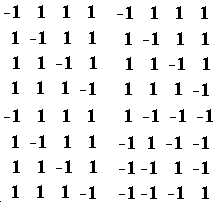Monday, June 27, 2022
HomeSoftware DevelopmentGenerate Hadamard matrix of given order

# Generate Hadamard matrix of given order

Hadamard matrix is a square matrix with the unique property that any two of its rows are orthogonal. In geometric terms that means that the two rows denote two perpendicular vectors and in combinatorial terms, it means that the two rows have matching elements in exactly half places and the other half elements are mismatching.

Some important properties of a Hadamard matrix are:

• The first order Hadamard matrix is {{1}}
• A Hadamard matrix contains only +1 and -1 as its elements.
• Any 2m order Hadamard matrix can be constructed using 2m-1 order Hadamard matrices in the following way. This facilitates the easy generation of the matrix if we know the lower-order matrices.

H2m
{ {H2m-1 ,  H2m-1}
{H2m-1, -H2m-1}}

Given a non-negative integer M, the task is to generate a Hadamard matrix of order 2M.

Examples:

Input: M = 2
Output:
1 1 1 1
1 -1 1 -1
1 1 -1 -1
1 -1 -1 1

Input: M = 3
Output:Approach: This is a simple implementation based problem. The idea is to use the above relation and iterate from order 1 to 2M to generate a Hadamard matrix of order  2M.

Follow the steps mentioned below to implement the idea.

• Calculate 2M (say N)and store it.
• Declare a matrix of order N
• Now initializing the 0th column 0th row element of the matrix as 1.
• Subsequently, run a loop that copies the top left quarter in the other quarters with a correct sign following the property of the Hadamard matrix as shown earlier in this article. The size of the matrix grows in each iteration and eventually reaches the order N
• Finally, display the matrix on the console.

Below is the implementation of the above approach.

## Java

 ` `  `import` `java.util.*;` ` `  `class` `GFG {` ` `  `    ``public` `static` `void` `generate(``int` `M)` `    ``{` `        ` `        ` `        ``int` `n = (``int``)Math.pow(``2``, M);` ` `  `        ` `        ``int``[][] hadamard = ``new` `int``[n][n];` ` `  `        ` `        ` `        ``hadamard[``0``][``0``] = ``1``;` `        ``for` `(``int` `k = ``1``; k < n; k += k) {` ` `  `            ` `            ` `            ``for` `(``int` `i = ``0``; i < k; i++) {` `                ``for` `(``int` `j = ``0``; j < k; j++) {` `                    ``hadamard[i + k][j]` `                        ``= hadamard[i][j];` `                    ``hadamard[i][j + k]` `                        ``= hadamard[i][j];` `                    ``hadamard[i + k][j + k]` `                        ``= -hadamard[i][j];` `                ``}` `            ``}` `        ``}` ` `  `        ` `        ``for` `(``int` `i = ``0``; i < n; i++) {` `            ``for` `(``int` `j = ``0``; j < n; j++) {` `                ``System.out.print(hadamard[i][j] + ``" "``);` `            ``}` `            ``System.out.println();` `        ``}` `    ``}` ` `  `    ` `    ``public` `static` `void` `main(String[] args)` `    ``{` `        ``int` `M = ``2``;` ` `  `        ` `        ``generate(M);` `    ``}` `}`
Output

```1 1 1 1
1 -1 1 -1
1 1 -1 -1
1 -1 -1 1
```

Time Complexity: O(2M)
Auxiliary Space: O(2M)

RELATED ARTICLES